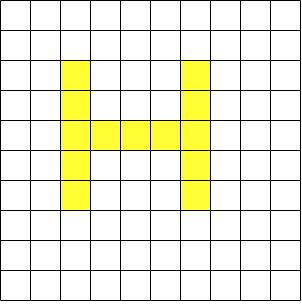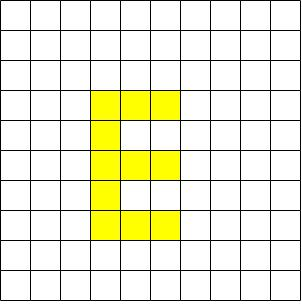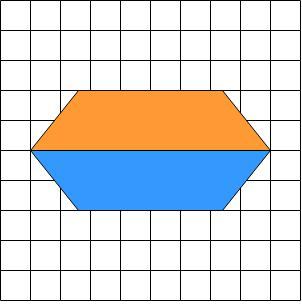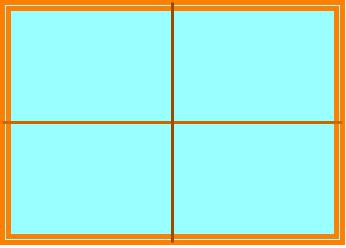# Enlargements and reductions with scale factors

### Enlargements and reductions with scale factors

When you are asked to make an object bigger or smaller, you will ask, "by how much?" In this case, scale factor can help answer the question. In this lesson, we will practice how to enlarge and reduce objects according to the scale factors given to us.
Basic Concepts:Ratios, Rates, Proportions,

#### Lessons

• Introduction
a)
What is a scale factor?

• 1.
Redraw the letters below using a scale factor of 2.
a)b)• 2.
Redraw the shape using a scale factor of $\frac{1}{4}$ .• 3.
The ratio of the length to the width of a window is 3:2. If the window is 8 m wide, what are the dimensions of the window that has a scale factor of:a)
0.5?

b)
2?

c)
3?